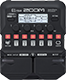# [Factory 1:7] RandyTrain

Discussion in 'Zoom G1/G1X Four' started by Tonelib, Apr 22, 2019.

1. [Factory 1:7] RandyTrainDevice: Zoom G1 Four
Firmware: 1.00

Name on device: RandyTrain
Optimized for: Phones/Speaker

Effects chain:This 80's metal sound uses DIST Plus.

Effect: "ZNR" (Dynamics), active - "yes"
"Detect" = EFXIN
"Depth" = 100
"Threshold" = 30
"Decay" = 0

Effect: "ParaEQ" (Filter), active - "yes"
"Frequency" = 800Hz
"Q" = 2.0
"Gain" = 8.5
"Volume" = 85

Effect: "DIST Plus" (Overdrive / Distortion), active - "yes"
"Gain" = 100
"Volume" = 100
"DryMx" = 0
"Comp" = ORG

Effect: "Gt GEQ 7" (Filter), active - "yes"
"100" = 7.0
"200" = 2.0
"400" = 3.0
"800" = 2.0
"1.6k" = 1.0
"3.2k" = 1.0
"6.4k" = -1.0
"VOL" = 74

Effect: "Room" (Reverb), active - "yes"
"PreD" = 3
"Decay" = 17
"Mix" = 63
"Tail" = Off

Patch Volume: 43